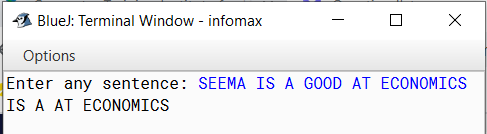# Java program to enter a sentence and print the words which starting with a vowel

Java program to enter a sentence and print the words which starting with a vowel

`Solution`

``````import java.util.*;
class Print_Vowel
{
public static void main(String args[])
{
Scanner sc=new Scanner(System.in);
System.out.print("Enter any sentence: ");
String s=sc.nextLine();
s = s+" ";
int l=s.length();
int pos=0;
char ch1, ch2;
String w;
for(int i=0; i<l; i++)
{
ch1 = s.charAt(i);
if(ch1 == ' ')
{
w = s.substring(pos,i); // extracting words one by one
ch2 = w.charAt(0);
if(ch2=='A' || ch2=='E' || ch2=='I' || ch2=='O' || ch2=='U' ||
ch2=='a' || ch2=='e' || ch2=='i' || ch2=='o' || ch2=='u')
{
System.out.print(w+"  ");
}
pos = i+1;
}
}
}
}``````
`Output`